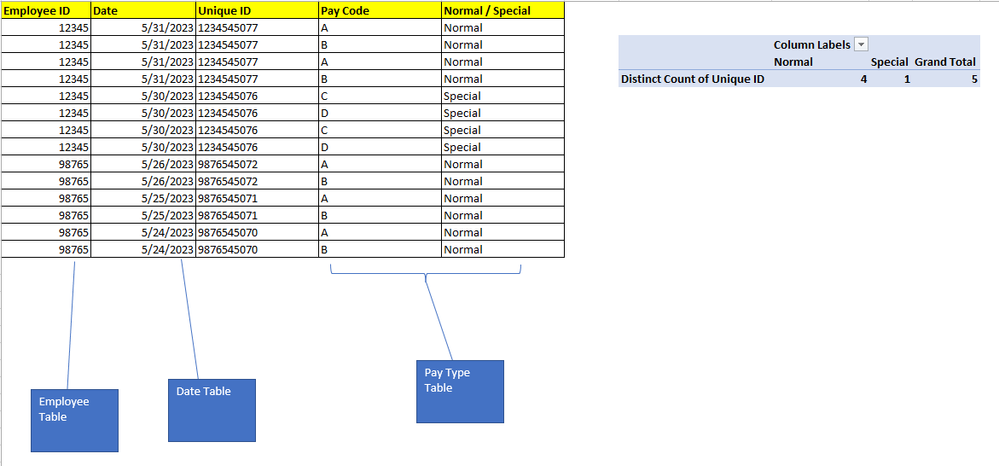cancel
Showing results for
Did you mean:Frequent Visitor

## Distinct Count using criteria from multiple tables

I am trying to get a unique count of rows joined from two different tables. I want to determine how many times an employee (Employee[Employee ID]) switched to a special pay code by day, (Date[Date]).

Below is an example of what I'm trying to do in the pivot table, but the Date and Employee ID data reside in different tables. I'm trying to create something like the "Unique ID" in Power BI, the excel formula is just a concatenation of Employee ID and Date.1 ACCEPTED SOLUTIONFrequent Visitor

The formula needed is the following:

Unique Count =

COUNTROWS(

SUMMARIZE(

RELATEDTABLE(Pay),

Employee[Employee ID],

'Date'[Date]

)

)Frequent Visitor

The formula needed is the following:

Unique Count =

COUNTROWS(

SUMMARIZE(

RELATEDTABLE(Pay),

Employee[Employee ID],

'Date'[Date]

)

)